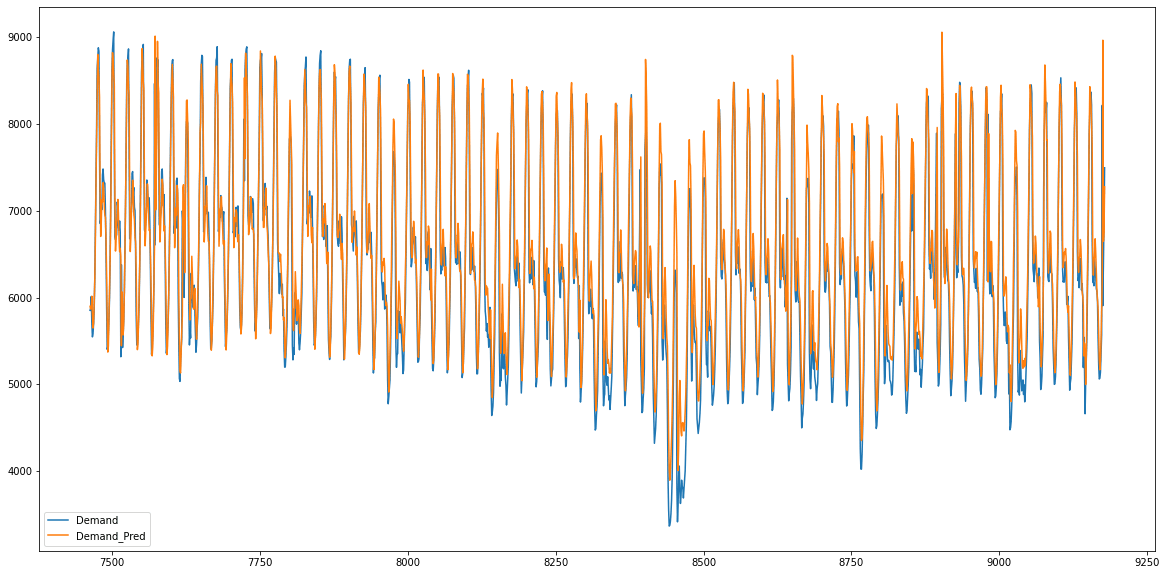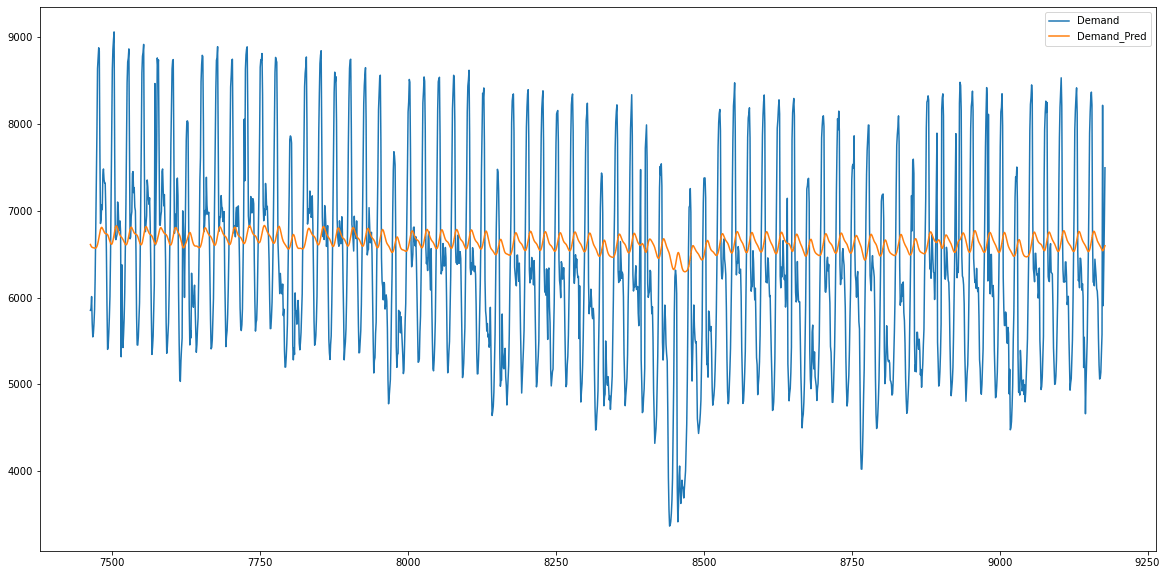# Building A Semi-Automated Electricity Demand Forecasting: A Bangladeshi Case Study

Thanks to advanced machine learning and deep learning algorithms, we see much demand- forecasting research in recent days. Due to the advent of renewable energy, demand forecasting is becoming one of the hot topics. Because of renewable resources’ intermittent nature, it is still not used as a base-load electricity source. So based on the demand forecasting data, we can allocate the energy resources efficiently.

This writing will show how I have implemented a semi-automated method to fetch the past electricity demand data and use them for future demand prediction. I have used the Power Grid Company of Bangladesh (PGCB) webpage, which provides hourly generation and demand data.

I used several python libraries for fetching the web page, data preparation, and time series analysis for this work. I hope you will find this helpful.

The first hurdle was to import the electricity demand data from the Power Grids website. For this, I have used the BeautifulSoup library.

At the beginning of this task, I tried the plain and simple code to fetch the website page, clean them for the necessary part, and then convert them to a data frame. But the problem is that the target webpage has several hundreds of pages. One single page contains only 51 observations. one day having 24 observations means one single page contains only 2 days of Electricity demand data.

Then used a for loop and put whole simple code inside the for loop and kept the l = [] out of the for loop so that the raw observation values can be stored inside the blank list.

After getting the DataFrame, there was a visible outlier;

As we can see from the above graph our demand data was never above 20000 MW. So we removed any data above 20000 MW. New graph looks like this.

After removing the outlier, Keras LSTM library is used to forecast. Thanks to AIEngineering’s detailed tutorial on LSTM.

First step was to normalize the data from 0 to 1. Here MinMaxScalar from sklearn library is used to do the normalization. The basic formula for normalization is as follows: y = (x – min) / (max – min)

As our dataset is a single feature dataset, we will not not use the datetime column as LSTM doesn’t need that data. LSTM will only need the feature data. So for LSTM data order is very important. That is reason at the time of train test split we set shuffle= False.

In lstm we have to set some parameters such as window length and batch size.

For window length I have considered 5 days of data. Grid demand data is updated in every single hour. So in a day it has 24 data point. In total it has 120 data points in five days. In each training instance we want to input 30 data so batch size is given as 30. Next number of feature is 1.

Next I will create a train generator where I passed the X_train and y_train through the TimeseriesGenerator with the window length as length and batch size data as input.

Next step is to create the stacked LSTM model with 20 epoch/iteration, and set to early stop if the val loss doesn’t improve in 2 iteration.epoch 20Original Demand value vs Demand Prediction

I also tried less iteration to check the graphepoch = 5

Finally the error has been calculated by Root Mean Squared of error term which is 480.